## Analyzing State-by-State Changes In Earthquake Frequency¶

In :
import pandas as pd
from matplotlib import pyplot as plt
%matplotlib inline


### Load and clean up data¶

The data was obtained from USGS here: http://earthquake.usgs.gov/earthquakes/search/. It was then run through a PostGIS database to determine the location of the epicenters by state, since USGS does provide specific state locations in its data.

In :
all_quakes = pd.DataFrame.from_csv("../data/earthquake_states.csv", index_col=None, parse_dates=["time", "updated"])


Some earthquakes within the U.S. bounding box don't have epicenters outside any state (e.g., the ocean).

In :
us_quakes = all_quakes.dropna(subset=["state"])

In :
state_counts = pd.DataFrame(us_quakes.state.value_counts())

In :
state_counts.columns = ["total_number_of_earthquakes"]

In :
state_counts

Out:
total_number_of_earthquakes
California 18108
Idaho 1231
Washington 973
Oklahoma 899
Montana 726
Wyoming 714
Hawaii 640
Utah 559
Oregon 376
Arizona 174
New Mexico 165
Arkansas 147
Texas 130
Missouri 92
Tennessee 81
Kansas 61
Illinois 61
Maine 36
Alabama 35
New York 35
Kentucky 33
South Carolina 32
South Dakota 29
Virginia 28
Ohio 22
Indiana 20
North Carolina 17
Pennsylvania 14
Georgia 14
New Hampshire 13
Massachusetts 10
New Jersey 10
Mississippi 9
West Virginia 8
Connecticut 5
Louisiana 5
Minnesota 5
North Dakota 3
Michigan 3
Maryland 2
Florida 1
Iowa 1

## Charting Earthquake Activity Over Time, By State¶

In :
ax = us_quakes[us_quakes["state"] == "Oklahoma"].set_index("time")["id"].resample("A", how="count").plot()
ax.set_title("Oklahoma Earthquake Count By Year")
pass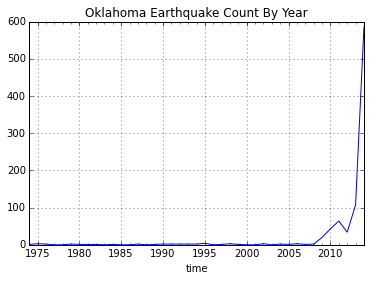In :
ax = us_quakes[us_quakes["state"] == "California"].set_index("time")["id"].resample("A", how="count").plot()
ax.set_title("California Earthquake Count By Year")
pass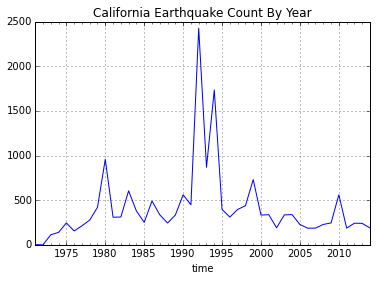In :
ax = us_quakes[us_quakes["state"] == "Texas"].set_index("time")["id"].resample("A", how="count").plot()
ax.set_title("Texas Earthquake Count By Year")
pass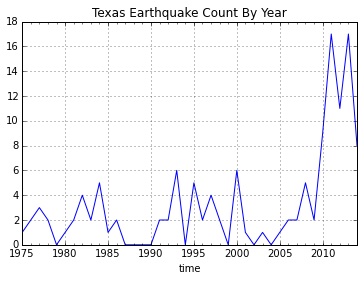In :
ax = us_quakes[us_quakes["state"] == "Ohio"].set_index("time")["id"].resample("A", how="count").plot(color="b")
ax.set_title("Ohio Earthquake Count By Year")
ax.set_ylim([0,5])
pass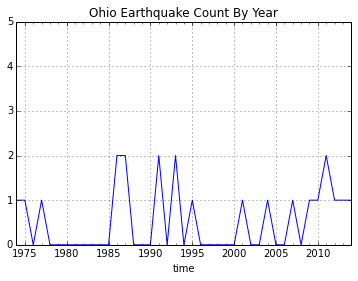In :
ax = us_quakes[us_quakes["state"] == "Colorado"].set_index("time")["id"].resample("A", how="count").plot()
ax.set_ylim([0,25])
pass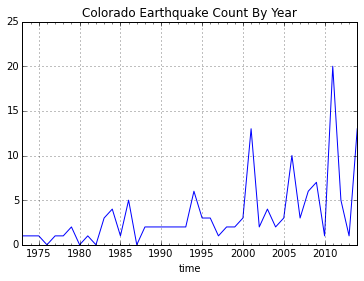In :
ax = us_quakes[us_quakes["state"] == "Tennessee"].set_index("time")["id"].resample("A", how="count").plot()
ax.set_title("Tennessee Earthquake Count By Year")
ax.set_ylim([0,10])
pass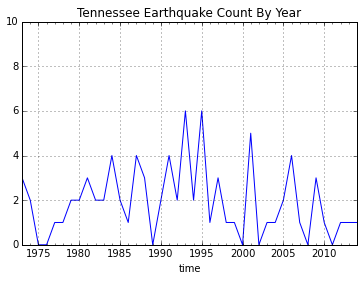In :
ax = us_quakes[us_quakes["state"] == "Kentucky"].set_index("time")["id"].resample("A", how="count").plot()
ax.set_title("Kentucky Earthquake Count By Year")
ax.set_ylim([0,10])
pass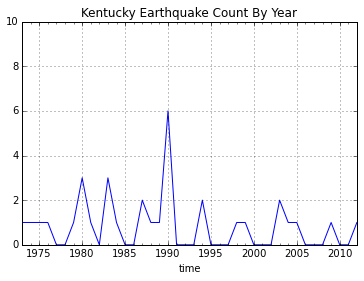In :
ax = us_quakes[us_quakes["state"] == "Kansas"].set_index("time")["id"].resample("A", how="count").plot()
ax.set_title("Kansas Earthquake Count By Year")
pass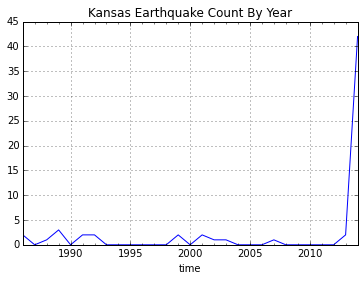In :
ax = us_quakes[us_quakes["state"] == "Arkansas"].set_index("time")["id"].resample("A", how="count").plot()
ax.set_title("Arkansas Earthquake Count By Year")
pass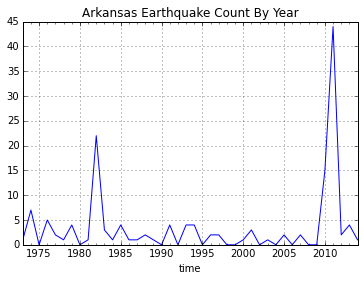The most recent complete year of earthquakes is 2014. Below, we compare 2005-2014 to the prior decade, 1995-2004.

In :
def quake_percentage_change(state):
by_year = pd.DataFrame(us_quakes[us_quakes["state"] == state].set_index("time")["id"].resample("AS", how="count"))
by_year["start"] = by_year.index
by_year["year"] = by_year["start"].apply(lambda x: x.year)
decade_05_14 = by_year[(by_year["year"] >= 2005) & (by_year["year"] <= 2014)]
decade_95_04 = by_year[(by_year["year"] >= 1995) & (by_year["year"] <= 2004)]
if total_95_04 != 0:
pct = round(100.0 * (total_05_14 - total_95_04) / total_95_04, 2)
else:
pct = None
return pct, total_05_14, total_95_04

In :
state_counts["name"] = state_counts.index

In :
state_counts["percentage_change"], state_counts["total_05-14"], state_counts["total_95-04"] =\
zip(*state_counts["name"].apply(lambda x: quake_percentage_change(x)))


In :
round(100.0 * (state_counts["total_05-14"].sum() - state_counts["total_95-04"].sum()) / state_counts["total_95-04"].sum(), 2)

Out:
-6.56

States with at least 5 earthquakes from 1995-2004 (sorted by percentage change decade-over-decade):

In :
state_counts[state_counts["total_95-04"] >= 5].sort("percentage_change", ascending=False)

Out:
total_number_of_earthquakes name percentage_change total_05-14 total_95-04
Oklahoma 899 Oklahoma 6042.86 860 14
Arkansas 147 Arkansas 677.78 70 9
Kansas 61 Kansas 650 45 6
Texas 130 Texas 252.38 74 21
Hawaii 640 Hawaii 233.33 240 72
Illinois 61 Illinois 200 15 5
Arizona 174 Arizona 195.45 65 22
Virginia 28 Virginia 160 13 5
New Mexico 165 New Mexico 46.15 57 39
South Dakota 29 South Dakota 14.29 8 7
Washington 973 Washington 8.28 183 169
Wyoming 714 Wyoming 5.41 156 148
Maine 36 Maine 0 6 6
Montana 726 Montana -2.6 150 154